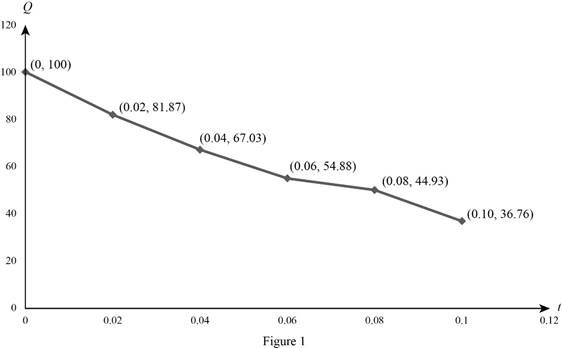# The exponential model for the charge.### Single Variable Calculus: Concepts...

4th Edition
James Stewart
Publisher: Cengage Learning
ISBN: 9781337687805### Single Variable Calculus: Concepts...

4th Edition
James Stewart
Publisher: Cengage Learning
ISBN: 9781337687805

#### Solutions

Chapter 3.4, Problem 77E

(a)

To determine

## To find: The exponential model for the charge.

Expert Solution

The exponential model for the charge Q(t)=100e10t.

### Explanation of Solution

Calculation:

Use the online graphing calculator and obtain the graph of an exponential model for the charge as shown below in Figure 1.The exponential model is Q(t)=Q0ekt (1)

From given table t=0 and Q(0)=100 then substitute in equation (1),

Q(0)=Q0e0100=Q0

Substitute Q0=100 and from given table t=0.02 and Q(0.02)=81.87 in equation (1),

Q(0.02)=100ek(0.02)81.87=100e0.02k81.87100=e0.02k0.8187=e0.02k

Take natural logarithm on both sides,

ln0.8187=ln(e0.02k)ln0.8187=0.02klne0.20.02=k                (Qlne=1)k=10

Substitute k=10 and Q0=100 in equation (1),

Q(t)=100e10t

Therefore, the exponential model for the charge Q(t)=100e10t.

(b)

To determine

### To estimate: The electric current when t=0.04s.

Expert Solution

The electric current when t=0.04s is Q(0.04)=670μA.

### Explanation of Solution

Calculation:

From part (a) the exponential model is Q(t)=100e10t.

Obtain the derivative Q(t).

Q(t)=ddt(Q(t))=ddt(100e10t)=100(10e10t)=1000e10t

Substitute t=0.04 in the above equation,

Q(0.04)=1000e10(0.04)=1000e0.4=1000(0.67)=670

Therefore, the electric current at t=0.04s is Q(0.04)=670 μA.

### Have a homework question?

Subscribe to bartleby learn! Ask subject matter experts 30 homework questions each month. Plus, you’ll have access to millions of step-by-step textbook answers!